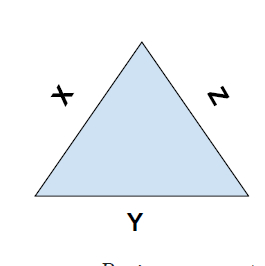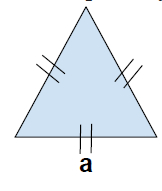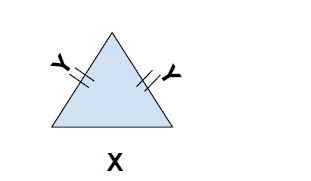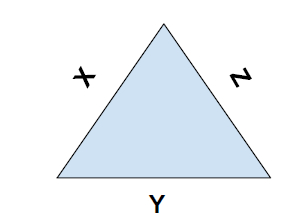# Find Perimeter of a triangle in C++

In this problem, we will see the perimeter of a triangle, formula for the perimeter of different types of triangle and program to find them.

Perimeter is defined as the total distance about the figure. Basically, it is the sum of all sides of the given figure.

Perimeter of a triangle

The perimeter of a triangle is the sum of all its three sides (triangle is a three sides figure).

Formula,

Perimeter = sum of all sidesPerimeter = x + y + z

Program to find the perimeter of a triangle,

## Example

Live Demo

#include <iostream>
using namespace std;
int calcPerimeter(int x, int y, int z ){
int perimeter = x + y + z;
return perimeter;
}
int main(){
int x = 5, y = 7, z = 8;
cout<<"The side of the triangle are \n";
cout<<"X = "<<x<<"\tY = "<<y<<"\tZ = "<<z<<endl;
cout<<"The perimeter of the triangle is "<<calcPerimeter(x, y, z);
return 0;
}

## Output

The side of the triangle are

X = 5 Y = 7 Z = 8
The perimeter of the triangle is 20

Perimeter of different type of triangles,

In mathematics, there are different types of triangle with some special properties. Though the basic formula of the perimeter remains the same, there are specific formulas of all types of triangle. Let’s see each of them.

## Equilateral Triangle

It is a special type of triangle in which all sides and angles are equal.Perimeter = 3*a

Program to find the area of equilateral triangle,

## Example

Live Demo

#include <iostream>
using namespace std;
int calcPerimeter(int a){
int perimeter = 3*a;
return perimeter;
}
int main(){
int a = 5;
cout<<"The side of the equilateral triangle are \n";
cout<<"a = "<<a<<endl;
cout<<"The perimeter of the triangle is "<<calcPerimeter(a);
return 0;
}

## Output

The side of the equilateral triangle are

a = 5
The perimeter of the triangle is 15

## Isosceles Triangle

It is a special type of triangle in which two sides are equal and the third one has different length.Perimeter = 2*X + Y

Program to find the perimeter of isosceles triangle,

## Example

Live Demo

#include <iostream>
using namespace std;
int calcPerimeter(int x, int y){
int perimeter = 2*x + y;
return perimeter;
}
int main(){
int x = 5, y = 8;
cout<<"The side of the Isosceles triangle are \n";
cout<<"X = "<<x<<"\tY = "<<y<<endl;
cout<<"The perimeter of the triangle is "<<calcPerimeter(x, y);
return 0;
}

## Output

The side of the Isosceles triangle are

X = 5 Y = 8
The perimeter of the triangle is 18

## Scalene triangle

It is a triangle whose all three sides are different.Perimeter = x + y + z